# Atomic Orbitals

Electron orbitals are the probability distribution of an electron in a atom or molecule.

10 April 2001: A minor update to Orbital Viewer has been posted.

 Overview A brief description of atomic orbitals (below). Orbital Viewer A program for drawing orbitals. This has many features, and comes in both a Windows version and a command-line interface version.Grand Table Lots of atomic orbitals, arrange by quantum number and shape. This table should make the orbital structure more obvious. I believe that it is the most complete orbital table anywhere.

## A Brief Overview

The electron orbitals presented here represent a volume of space within which an electron would have a certain probability of being based on particular energy states and atoms. For example, in a simple lowest-energy state hydrogen atom, the electrons are most likely to be found within a sphere around the nucleus of an atom. In a higher energy state, the shapes become lobes and rings, due to the interaction of the quantum effects between the different atomic particles. In addition to technical merits, they make pretty pictures.

The shape of the orbital depends on many factors. The most important are the quantum numbers associated with the particular energy state. These are n, the principal quantum number, l, the orbital quantum number, and m, the angular momentum quantum number. The following table shows some of these shapes. Also available is the Grand Table, showing many, many more orbitals in six different organizations.

 n=1,l=0 n=2,l=0 n=2,l=1 n=3,l=0 n=3,l=1 n=3,l=2 n=4,l=0 n=4,l=1 n=4,l=2 n=4,l=3 m=0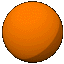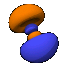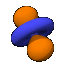m=1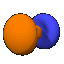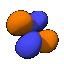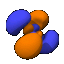m=2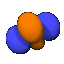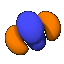m=3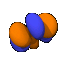These shapes continue on infinitely, getting ever more lobes or rings on them. Although the l=0, m=0 orbitals look like simple spheres, regardless of n value, this is not actually the case. To the right is a cutaway of a 4s0 (n=4, l=0, m=0) oribital, showing that it is really concentric spheres.

A note about the drawings: All of the pictures on this page were produced by the program Orbital Viewer, written by myself. The blue color indicates a positive phase, while the orange color indicates a negative phase, with the phase taken as defined by Condon and Shortley. The colors become important when molecular orbitals are computed.So far, all of the pictures have been of electron orbitals associated with a single atom. Molecules can become much more complicated. When two atoms are within a certain proximity of each other, the orbital probabilities can either reinforce each other or cancel each other out. If the phase is the same sign (the same color), the probabilities are reinforced. To the right is a picture of the bonding orbit for H2O (water).

If you wish to see more atomic orbitals, here are four MPEG video files of orbitals rotating. They are 5D0.MPG, 4F0.MPG, 5F0.MPG, and 5G4.MPG. These range from 500 to 750 kb.